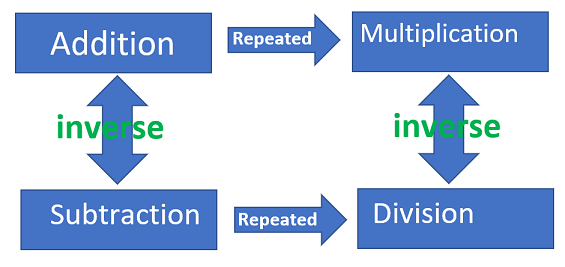# Four Basic Math Rules#What are the Four Basic Rules of Mathematics

An online basic mathematics rules printable

### Four Rules of Math

The concepts of addition, subtraction, multiplication and division are complex abstract ideas which are interrelated.positive + positive = (add) positive

Example: 2 + 1 = 3

negative + negative = (add) negative

Example: −3+ (−5) = −8

negative + positive = (subtract)

Example: 2 + (−10) = −8

Take sign of number with largest absolute value

Example: −14 + 16 = 2

Note: −(−7) means take the opposite of (−7) = 7

## 2. Subtraction Rules:

Change all subtraction to addition and take the opposite sign of the following number then follow the addition rules.

Example: −7 −(9)

means −7 +(−9) = -16

Example: −3 −(−10)

−3 + (10) = 7

Example:

−8 + (−9) −(−1)−2 (change all subtraction signs)

−8 + (−9)+ (1) + (−2) (do addition and subtraction from left to right)

−17 +(1)+ (−2)

−16 +(−2) = −18

Adding and subtracting with negative numbers:

When adding and subtracting positive and negative numbers it is useful to remember the follow-ing rules.

If the operation and the sign are the same, they work like adding a (positive) number,so that

works like ++

If the operation and the sign are different, they work like subtracting a (positive) number, sothat

+ works like −+

## 3 and 4. Multiplication / Division Rules:

The rules for multiplication and division are the same.

positive (✗ or ÷) positive = positive

Example: 10 ÷ 2 = 5

negative (✗ or ÷) negative = positive

Example: −4 ✗(−3) = 12

negative (✗ or ÷) positive = negative

Example: 18 ÷ (−2) −9Multiplying and dividing with negative numbers:

When multiplying pairs of positive and negative numbers it is helpful to remember the followingrules:When the signs of the numbers are the same the answer is a positive number.

(+) ✗ (+) , answer is (+)

(−) ✗ (−) , answer is (+)

(+) ÷ (+) , answer is (+)

(−) ÷ (−) , answer is (+)

When the signs of the numbers are different the answer is a negative number.

(+) ✗ (−) , answer is (−)

(−) ✗ (+) , answer is (−)

(+) ÷ (−) , answer is (−)

(−) ÷ (+) , answer is (−)##Age Calculators ►

Horse Age Calculator , Cow Age Calculator , Cat Age Calculator , Dog Age Calculator, Human Age Calculator 100 Days Old Calculator Date Difference Calculator